Orginal Article

# 多柔体系统数值分析的模型降噪方法

1大连理工大学工程力学系, 大连 116023
2大连理工大学盘锦校区海洋科学与技术学院, 辽宁盘锦 124221;

# MODEL SMOOTHING METHODS IN NUMERICAL ANALYSIS OF FLEXIBLE MULTIBODY SYSTEMS

1Department of Engineering Mechanics, Dalian University of Technology, Dalian 116023, China
2School of Ocean Science and Technology, Dalian University of Technology, Panjin 124221, Liaoning, China ;

Abstract

Dynamic equations of flexible multibody systems are usually a set of stiff differential equations. At present, the common numerical method for solving the stiff differential equations filters out the high frequency by using the numerical damping. The computational efficiency of this method is still unsatisfactory. In order to reduce the stiffness of dynamic equations of flexible multibody systems so greatly that the equations can be solved by regular ordinary differential equation (ODE) solvers such as MATLAB ODE45 solver, methods of filtering high frequency vibrations during the process of modeling are studied. Stresses of flexible bodies are homogenized by their mean value over a time interval from now to a short time later. The homogenized stress is then employed to replace its origin when computing the virtual deformation power. In this way, the obtained model of the flexible multibody system will not contain harmful high frequency elastic vibrations. The range of frequencies can be controlled by the length of the time interval used to homogenize stresses. As validated by the numerical examples in this paper, the precision and efficiency of the proposed method are comparable to some stiff ODE solvers. Moreover, it works well when the stiff ODE solver fails to give correct solutions in a reasonable time. Comparisons of numerical examples show that the proposed method can be a new available approach to numerical analysis of flexible multibody systems.

Keywords： flexible multibody systems ; model smoothing ; stiff ODEs ; virtual deformation power ; principle of virtual power

0

Qi Zhaohui, Cao Yan, Wang Gang. MODEL SMOOTHING METHODS IN NUMERICAL ANALYSIS OF FLEXIBLE MULTIBODY SYSTEMS[J]. Acta Mechanica Sinica, 2018, 50(4): 863-870 https://doi.org/10.6052/0459-1879-18-111

$||f(t,y2)-f(t,y1)||L||y2-y1||$

Lipschitz常数越大, 数值求解的难度也越大[14,15,16]. 多柔体系统中物体的高频振荡弹性运动可使系统动力学方程的Lipschitz常数高达 $106$量级以上. 因而, 在多柔体系统数值分析中有一个物理上十分不合理的现象: 当一个柔性体的弹性模量很高, 物体的运动趋近于相对简单的刚体运动时, 数值分析的难度反而大幅度提升. 分析多柔体系统时需要采用刚性微分方程求解器, 否则数值解将难以在合理的时间内获得. 刚性微分方程求解方法可划分为两类[17,18,19,20,21]: 一类以标准一阶微分方程为研究对象, 其主要方法包括: BDF 方法、隐式Runge-Kutta方法、Rosenbrock方法等; 另一类以二阶微分方程为研究对象, 其主要方法包括: Newmark方法、Wilson- $θ$方法、HHT方法、广义 $α$方法等. 由于多柔体系统动力学方程属于微分代数混合方程, 还需要将这些方法与DAE解法相结合. 其主要方法有[22,23,24,25,26,27,28,29]: HHT 双循环隐式积分法、BDF双循环隐式积分法、广义 $α$投影方法以及新型广义 $α$方法等. 所有这些方法都有一个共同点: 采用数值阻尼滤除了系统中的高频分量. 正是因为滤除了高频分量, 才能使积分步长远远大于最小振动周期时不引起积累误差的放大传播. 既然系统模型中的高频分量在求解时也要被滤除, 如何在建模时就滤除这些高频分量是非常值得研究的课题.

## 1 多柔体系统数值求解的困难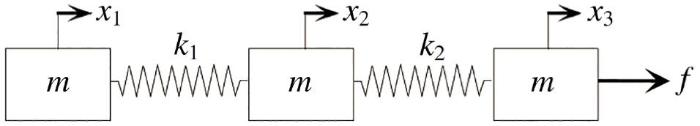Fig.1   Spring-mass system

$mẍ1+k(x1-x2)=0$

$mẍ2+2kx2-k(x1+x3)=0$

$mẍ3+kx3-kx2=f$

$x0=12a0t2$

$x1=x0-a1(1-cosω1t)+a2(1-cosω2t)$

$x2=x0-2a2(1-cosω2t)$

$x3=x0+a1(1-cosω1t)+a2(1-cosω2t)$

$ẍ1=a0+12a0(cosω2t-3cosω1t)$

$ẍ2=a0-a0cosω2t$

$ẍ3=a0+12a0(3cosω1t+cosω2t)$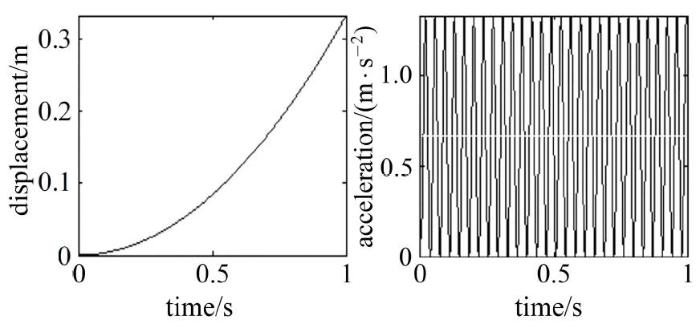Fig.2   Displacement and acceleration of a flexible body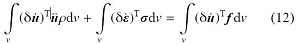$\begin{equation*}\textbf{{σ}}_\tau\approx\textbf{{σ}}_t+(\tau-t)\dot{\textbf{{σ}}}_t+(\tau-t)^2\ddot{\textbf{{σ}}}_t/2\tag*{(13)}\end{equation*}$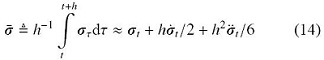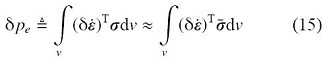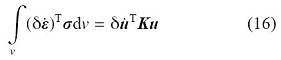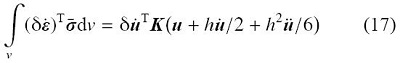$Mu+Ku=F$(18)

$(M+h2K/6)u+hKu/2+Ku=F$(19)

$\begin{equation*}(\textbf{{K}}-\omega^2\textbf{{M}})\textbf{{ν}}=\textbf{0}\tag*{(20)}\end{equation*}$

$ẅi+ωi2wi=fi,i=1,2,⋅⋅⋅,n$

$(1+h26ωi2)w̅̈i+ωi2(h2w̅̇i+w̅i)=fi,i=1,2,⋅⋅⋅,n$

$λω≜(1+h2ωi2/6)-1(1+5h2ωi2/48)12$

$λa≜(1+h2ωi2/6)-1$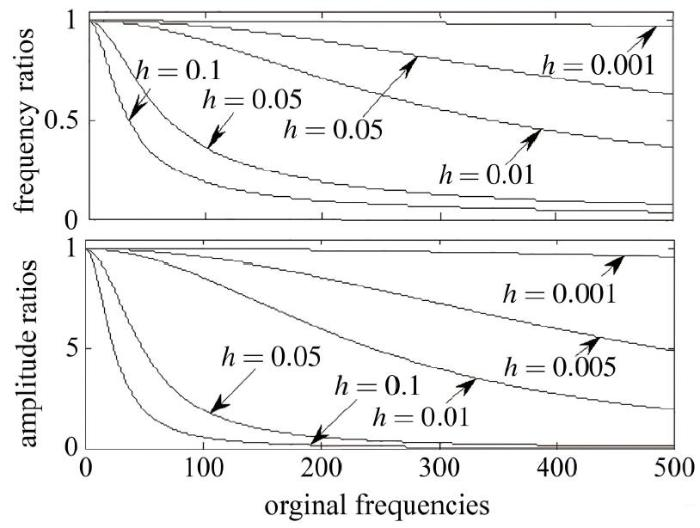Fig.3   Amplitudes and frequencies ratios

## 3 数值算例

Table 1   System parameters

$m$/kg$k1$/(N$⋅$m$-1)$$k2$/(N$⋅$m$-1)$$f$/N
$107$$108$$20$

$x1=x2=x3=t2/3$

Table 2   Comparisons of precision and efficiency ($εr=1.0×10-4$, $εa=1.0×10-6$)

SolversMaximum absolute errorTime/s
ODE45$1.5×10-5$376.9
ODE15s$2.3×10-5$884.4
MS$_$ODE45$_$0.1$1.6×10-5$0.27

Table 3   Comparisons of precision and efficiency ($εr=1.0×10-2$, $εa=1.0×10-3$)

SolversMaximum absolute errorTime/s
ODE45$8.8×1011$376.7
ODE15s$1.6×10-4$0.24
MS$_$ODE45$_$0.1$2.2×10-3$0.24

$x1=t2/3-2[1-cos(103t)]/900$

$x2=x3=t2/3+[1-cos(103t)]/900$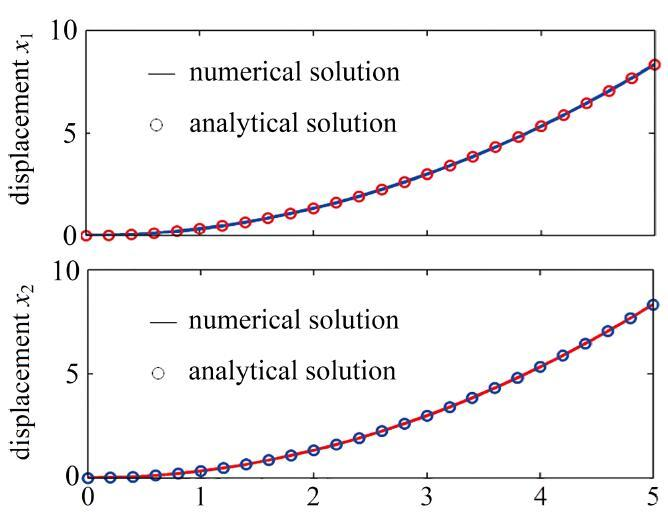Fig.4   Comparisons of numerical displacements and their analytical solution

Table 4   Comparisons of precision and efficiency (rigid-flexible coupling system)

SolversMaximum absolute errorTime/s
MS$_$ODE45$_$0.1$2.34×10-3$0.31
MS$_$ODE45$_$0.01$2.16×10-3$1.06
MS$_$ODE45$_$0.001$6.83×10-4$5.80
MS$_$ODE15s$_$0.001$2.35×10-2$0.57

$ε̅=εt+12hε̇t+16h2ε̈tκ̅=κt+12hκ̇t+16h2κ̈t$

$fint=Ke瀼$

$Ke=EAL000EIL/3EIL/60EIL/6EIL/3$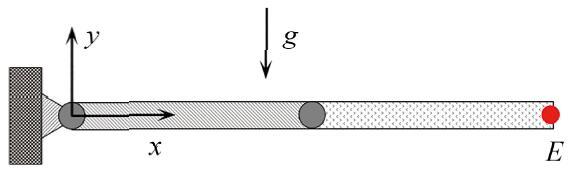Fig.5   Free-falling flexible double pendulum mechanism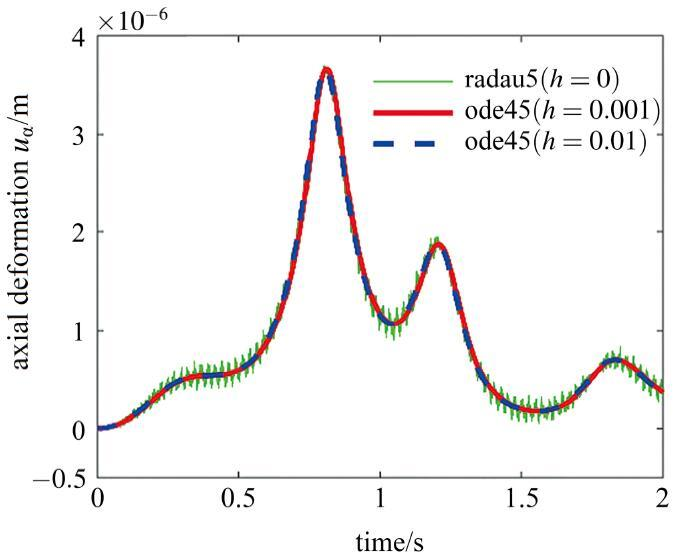Fig.6   Axial deformation of the end point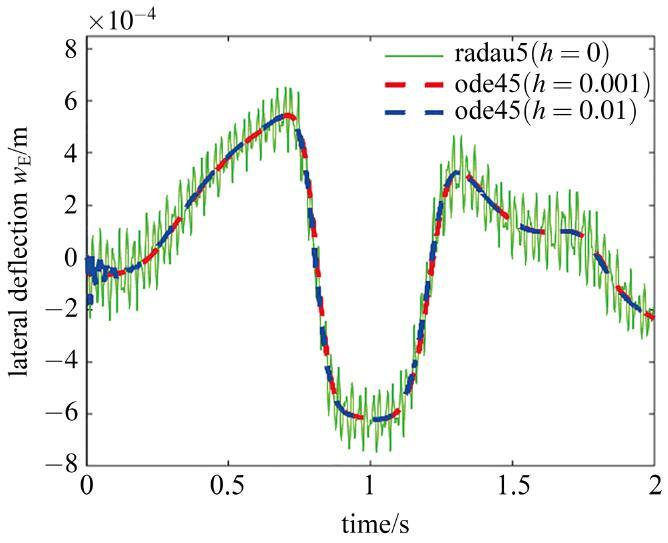Fig.7   Lateral deflection of the end point

Table 5   Efficiency comparison (flexible double pendulum mechanism)

SolversTime/s
MS$_$ODE45$_$0.0114
MS$_$ODE45$_$0.001116
MS$_$ODE45- -

## 4 结 论

The authors have declared that no competing interests exist.

## 参考文献 原文顺序 文献年度倒序 文中引用次数倒序 被引期刊影响因子

  田强, 刘铖, 李培等. 多柔体系统动力学研究进展与挑战. 动力学与控制学报, 2017, 15(5): 385-405 (Tian Qiang, Liu Cheng, Li Pei, et al.Advances and challenges in dynamics of flexible multibody systems.Journal of Dynamics and Control, 2017, 15(5): 385-405 (in Chinese))  王琪, 庄方方, 郭易圆等. 非光滑多体系统动力学数值算法的研究进展. 力学进展, 2013, 43(1): 101-111 (Wang Qi, Zhuang Fangfang, Guo Yiyuan, et al.Advances in the research on numerical methods for non-smooth dynamics of multibody systems.Advances in Mechanics, 2013, 43(1): 101-111 (in Chinese))  刘才山, 陈滨, 彭瀚等. 多体系统多点碰撞接触问题的数值求解方法. 动力学与控制学报, 2003, 1(1): 59-65 (Liu Caishan, Chen Bin, Peng Han, et al.Numerical resolution of multibody systems with multiple contact/impact points.Journal of Dynamics and Control, 2003, 1(1): 59-65 (in Chinese))  姚文莉, 岳嵘. 有争议的碰撞恢复系数研究进展. 振动与冲击, 2015, 34(19): 43-48 (Yao Wenli, Yue Rong.Advance in controversial restitution coefficient study for impact problems.Journal of Vibration and Shock, 2015, 34(19): 43-48 (in Chinese))  Stechlinski PG, Barton PI.Dependence of solutions of nonsmooth differential-algebraic equations on parameters.Journal of Differential Equations, 2017, 262(3): 2254-2285  Dhamacharoen A.Efficient numerical methods for solving differential algebraic equations.Journal of Applied Mathematics ＆ Physics, 2016, 4(1): 1-9  Petzold L.Differential/algebraic equations are not ODE’S.Siam Journal on Scientific ＆ Statistical Computing, 2012, 3(3): 367-384  Shampine LF, Reichelt MW, Kierzenka JA.Solving index-I DAEs in MATLAB and simulink.Siam Review, 1999, 41(3): 538-552  Gear CW, Petzold LR.ODE methods for the solution of differential /algebraic systems.Siam Journal on Numerical Analysis, 1984, 21(4): 716-728  Haddouni M, Acary V, Garreau S, et al.Comparison of several formulations and integration methods for the resolution of DAEs formulations in event-driven simulation of nonsmooth frictionless multibody dynamics.Multibody System Dynamics, 2017, 41(3): 201-231  Marques F, Souto A P, Flores P.On the constraints violation in forward dynamics of multibody systems.Multibody System Dynamics, 2016, 39(4): 1-35  Schweizer B, Lu D, Li P.Co-simulation method for solver coupling with algebraic constraints incorporating relaxation techniques.Multibody System Dynamics, 2016, 36(1): 1-36  潘振宽, 赵维加, 洪嘉振等. 多体系统动力学微分/代数方程组数值方法. 力学进展, 1996, 26(1): 83-96 (Pan Zhenkuan, Zhao Weijia, Hong Jiazhen, et al.On numerical algorithms for differential/algebraic equations of motion of multibody system.Advances in Mechanics, 1996, 26(1): 26-40 (in Chinese))  袁新鼎. 刚性常微分方程初值问题的数值解法. 北京: 科学出版社, 1987 (Yuan Xinding.Numerical Methods for Solving Initial Value Problems of Stiff Ordinary Differential Equations. Beijing: Science Press, 1987 (in Chinese))  程正兴. 数值逼近与常微分方程数值解. 西安: 西安交通大学出版社, 2000 (Cheng Zhengxing.Numerical Approximation and Numerical Solution of Ordinary Differential Equation. Xi’an: Xi’an Jiaotong University Press, 2000 (in Chinese))  Hairer E, Lubich C.Numerical Analysis of Ordinary Differential Equations. Berlin, Heidelberg: Springer 2015  Ibrahim Z, Nasir NM, Othman K, et al.Adaptive order of block backward differentiation formulas for stiff ODEs.Numerical Algebra, 2017, 7(1): 95-106  El-Zahar ER, Habib HM, Rashidi MM, et al.A comparison of explicit semi-analytical numerical integration methods for solving stiff ODE systems.American Journal of Applied Sciences, 2015, 12(5): 304-320  Ariel G, Engquist B, Tsai R.A multiscale method for highly oscillatory ordinary differential equations with resonance.Mathematics of Computation, 2009, 78(266): 929-956  Tokman M.Efficient integration of large stiff systems of ODEs with exponential propagation iterative (EPI) methods.Journal of Computational Physics, 2006, 213(2): 748-776  Meijaard JP.Application of Runge-Kutta-Rosenbrock methods to the analysis of flexible multibody systems.Multibody System Dynamics, 2003, 10(3): 263-288  Shabana AA, Hussein BA.A two-loop sparse matrix numerical integration procedure for the solution of differential/algebraic equations: Application to multibody systems.Journal of Sound ＆ Vibration, 2009, 327(3): 557-563  Yen J, Petzold L, Raha S.A time integration algorithm for flexible mechanism dynamics: the DAE α-method.Computer Methods in Applied Mechanics and Engineering, 1998, 158(3): 341-355  Arnold M, Brüls O.Convergence of the generalized-α, scheme for constrained mechanical systems.Multibody System Dynamics, 2007, 18(2): 185-202  丁洁玉, 潘振宽. 多体系统动力学微分‒代数方程广义<inline-formula><mml:math xmlns:mml="http://www.w3.org/1998/Math/MathML" id="Mml184-0459-1879-50-4-863"><mml:mo>-</mml:mo><mml:mi>α</mml:mi></mml:math></inline-formula>投影法. 工程力学, 2013, 30(4): 380-384 (Ding Jieyu, Pan Zhenkuan. generalized<inline-formula><mml:math xmlns:mml="http://www.w3.org/1998/Math/MathML" id="Mml185-0459-1879-50-4-863"><mml:mo>-</mml:mo><mml:mi>α</mml:mi></mml:math></inline-formula> projection method for differential-algebraic equations of multibody dynamics.Engineering Mechanics, 2013, 30(4): 380-384 (in Chinese))  郭晛, 章定国, 陈思佳. Hilber-Hughes-Taylor<inline-formula><mml:math xmlns:mml="http://www.w3.org/1998/Math/MathML" id="Mml186-0459-1879-50-4-863"><mml:mo>-</mml:mo><mml:mi>α</mml:mi></mml:math></inline-formula> 法在接触约束多体系统动力学中的应用. 物理学报, 2017, 66(16): 144-154 (Guo Xian, Zhang Dingguo, Chen Sijia.Application of Hilber-Hughes-Taylor<inline-formula><mml:math xmlns:mml="http://www.w3.org/1998/Math/MathML" id="Mml187-0459-1879-50-4-863"><mml:mo>-</mml:mo><mml:mi>α</mml:mi></mml:math></inline-formula> method to dynamics of flexible multibody system with contact and constraint.Acta Physica Sinica, 2017, 66(16): 144-154 (in Chinese))  姚廷强, 迟毅林, 黄亚宇. 柔性多体系统动力学新型广义<inline-formula><mml:math xmlns:mml="http://www.w3.org/1998/Math/MathML" id="Mml188-0459-1879-50-4-863"><mml:mo>-</mml:mo><mml:mi>α</mml:mi></mml:math></inline-formula>数值分析方法. 机械工程学报, 2009, 45(10): 53-60 (Yao Tingqiang, Chi Yilin, Hu Yayu.New generalized-α algorithms for multibody dynamics.Journal of Mechanical Engineering, 2009, 45(10): 53-60 (in Chinese))  郭晛, 章定国. 柔性多体系统动力学典型数值积分方法的比较研究. 南京理工大学学报(自然科学版), 2016, 40(6): 726-733 (Guo Xian, Zhang Dingguo.Comparative study of typical numerical integration methods of flexible multi-body systems dynamics.Journal of Nanjing University of Science and Technology, 2016, 40(6): 726-733 (in Chinese))  Banerjee A.Flexible Multibody Dynamics: Efficient Formulations and Applications. John Wiley ＆ Sons, Inc., 2016  洪嘉振. 计算多体系统动力学. 北京: 高等教育出版社, 1999 (Hong Jiazhen.Computational Dynamics of Multibody Systems. Beijing: Higher Education Press, 1999 (in Chinese))  齐朝晖. 多体系统动力学. 北京: 科学出版社, 2008 (Qi Zhaohui.Dynamics of Multibody Systems. Beijing: Science Press, 2008 (in Chinese))  张志刚, 齐朝晖, 吴志刚. 一种基于应变插值大变形梁单元的刚‒柔耦合动力学分析. 振动工程学报, 2015, 28(3): 337-344 (Zhang Zhigang, Qi Zhaohui, Wu Zhigang.Rigid-flexible dynamics analysis of a large deformation beam element based on interpolation of strains.Journal of Vibration Engineering, 2015, 28(3): 337-344 (in Chinese))/

 〈〉